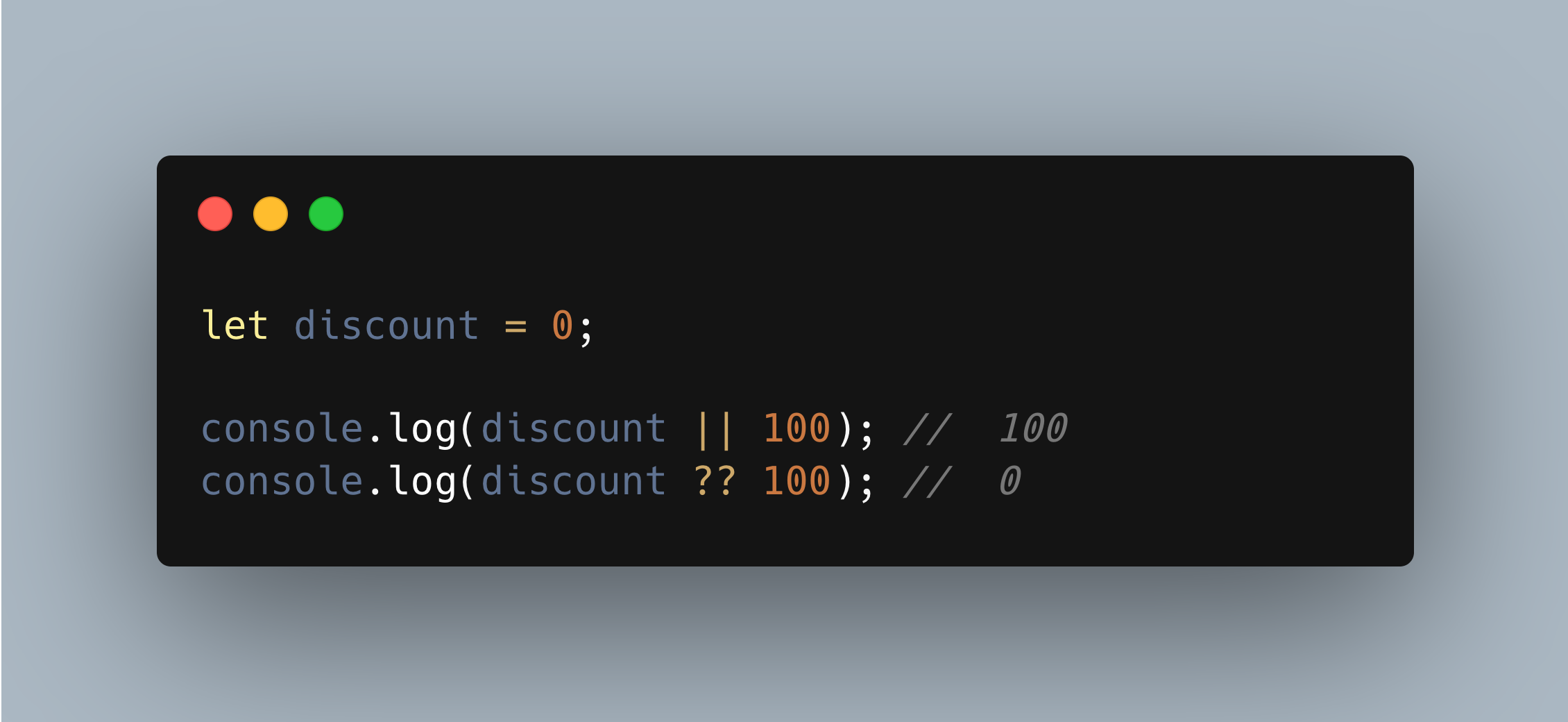{{mmanishh}}

# ?? vs || Operator in Javascript

Jan 16, 2023
2 minutesThere are two operators `??` (Nullish coalescing operator) and `||` (OR Operator) which is confusing though their usage is almost similar but there is a subtle difference between the two which we will be going through in this post.

# The Difference

The important difference between them is that:

``````|| returns the first truthy value
?? returns the first defined value
``````

The result of `a || b` is:

• if the Boolean type conversion value of a is true i.e Boolean(a)=true, then a,
• if the Boolean type conversion value of b is false i.e Boolean(a)=false, then b,

The result of `a ?? b` is:

• if a is defined, then a,
• if a isn’t defined, then b.

## What does that mean?

Let’s see this through different examples:

Example 1

``````let discount = 0;

console.log(discount || 100); // 100
console.log(discount ?? 100); // 0
``````

Here, the discount is 0 so

• `||` will result to 100 since `Boolean(0) = false`
• `??` will result in 0 because 0 is defined

Example 2

``````let discount = 1;

console.log(discount || 100); // 1
console.log(discount ?? 100); // 1
``````

Here, the discount is 1 so

• `||` will result to 1 since `Boolean(1) = true`
• `??` will result in 1 because 1 is defined

Example 3

``````let discount = null;

console.log(discount || 100); // 100
console.log(discount ?? 100); // 100
``````

Here, the discount is null so

• `||` will result in 100 because it evaluates null as false since `Boolean(null) = false`
• `??` will result in 100 because null is undefined

Example 4

``````let discount = "";

console.log(discount || 100); // 100
console.log(discount ?? 100); // ""
``````

Here, the discount is an empty string so

• `||` will result in 100 because it evaluates an empty string as false since `Boolean("") = false`
• `??` will result in “” because an empty string is defined in JS

## Using ?? with && or ||

Javascript forbids using with `??`, `||` and `&&` together. It will raise Syntax Error.

``````let x = 1 || 2 ?? 3; // Syntax error
``````

However you can use explicit parentheses to work around it:

``````let x = (1 || 2) ?? 3; // 1
``````

## Conclusion

• The nullish coalescing operator ?? provides a short way to choose the first “defined” value from a list.
• It’s used to assign default values to variables:
``````discount = discount ?? 10 // assigns discount as 10 if its null/undefined
``````
• The OR operator || provides a short way to choose the first truthy value from a list.
• You cannot use operators `??` with `&&` or `||` together. You need to use explicit parantheses